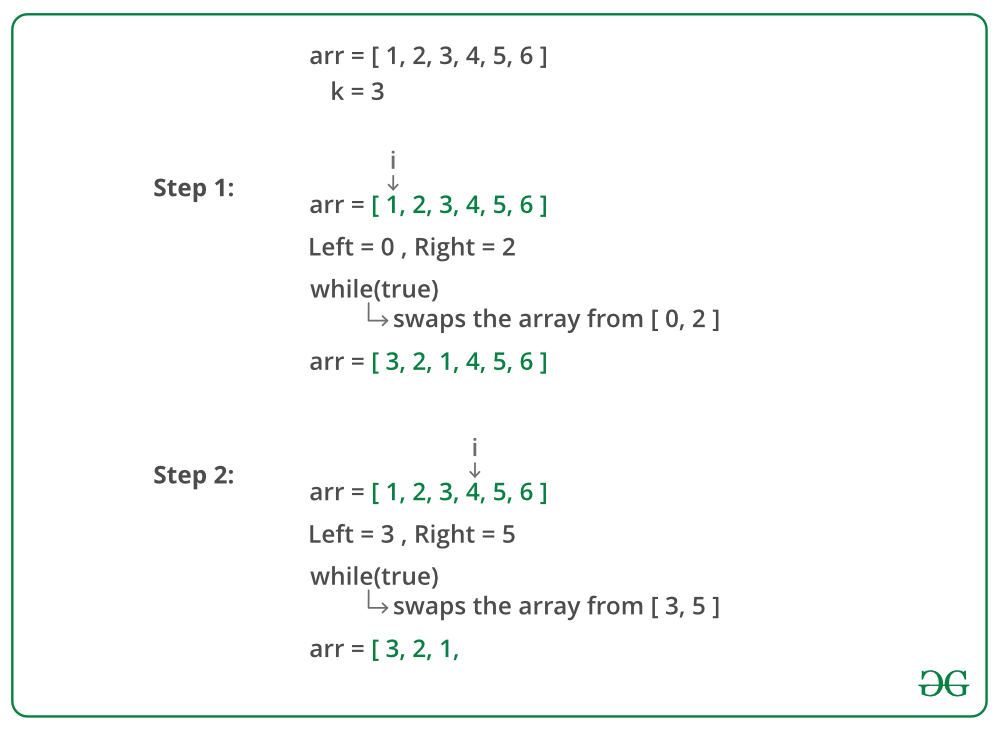# Reverse an array in groups of given size

• Difficulty Level : Easy
• Last Updated : 01 Jun, 2022

Given an array, reverse every sub-array formed by consecutive k elements.

Examples:

Input:
arr = [1, 2, 3, 4, 5, 6, 7, 8, 9]
k = 3
Output:
[3, 2, 1, 6, 5, 4, 9, 8, 7]

Input:
arr = [1, 2, 3, 4, 5, 6, 7, 8]
k = 5
Output:
[5, 4, 3, 2, 1, 8, 7, 6]

Input:
arr = [1, 2, 3, 4, 5, 6]
k = 1
Output:
[1, 2, 3, 4, 5, 6]

Input:
arr = [1, 2, 3, 4, 5, 6, 7, 8]
k = 10
Output:
[8, 7, 6, 5, 4, 3, 2, 1]

Approach: Consider every sub-array of size k starting from the beginning of the array and reverse it. We need to handle some special cases. If k is not multiple of n where n is the size of the array, for the last group we will have less than k elements left, we need to reverse all remaining elements. If k = 1, the array should remain unchanged. If k >= n, we reverse all elements present in the array.

Below image is a dry run of the above approach:Below is the implementation of the above approach:

## C++

 `// C++ program to reverse every sub-array formed by` `// consecutive k elements` `#include ` `using` `namespace` `std;`   `// Function to reverse every sub-array formed by` `// consecutive k elements` `void` `reverse(``int` `arr[], ``int` `n, ``int` `k)` `{` `    ``for` `(``int` `i = 0; i < n; i += k)` `    ``{` `        ``int` `left = i;`   `        ``// to handle case when k is not multiple of n` `        ``int` `right = min(i + k - 1, n - 1);`   `        ``// reverse the sub-array [left, right]` `        ``while` `(left < right)` `            ``swap(arr[left++], arr[right--]);`   `    ``}` `}`   `// Driver code` `int` `main()` `{` `    ``int` `arr[] = {1, 2, 3, 4, 5, 6, 7, 8};` `    ``int` `k = 3;`   `    ``int` `n = ``sizeof``(arr) / ``sizeof``(arr);`   `    ``reverse(arr, n, k);`   `    ``for` `(``int` `i = 0; i < n; i++)` `        ``cout << arr[i] << ``" "``;`   `    ``return` `0;` `}`

## Java

 `// Java program to reverse every sub-array formed by` `// consecutive k elements` `class` `GFG {` `    `  `    ``// Function to reverse every sub-array formed by` `    ``// consecutive k elements` `    ``static` `void` `reverse(``int` `arr[], ``int` `n, ``int` `k)` `    ``{` `        ``for` `(``int` `i = ``0``; i < n; i += k)` `        ``{` `            ``int` `left = i;` `    `  `            ``// to handle case when k is not multiple` `            ``// of n` `            ``int` `right = Math.min(i + k - ``1``, n - ``1``);` `            ``int` `temp;` `            `  `            ``// reverse the sub-array [left, right]` `            ``while` `(left < right)` `            ``{` `                ``temp=arr[left];` `                ``arr[left]=arr[right];` `                ``arr[right]=temp;` `                ``left+=``1``;` `                ``right-=``1``;` `            ``}` `        ``}` `    ``}` `    `  `    ``// Driver method` `    ``public` `static` `void` `main(String[] args)` `    ``{` `        `  `        ``int` `arr[] = {``1``, ``2``, ``3``, ``4``, ``5``, ``6``, ``7``, ``8``};` `        ``int` `k = ``3``;` `    `  `        ``int` `n = arr.length;` `    `  `        ``reverse(arr, n, k);` `    `  `        ``for` `(``int` `i = ``0``; i < n; i++)` `            ``System.out.print(arr[i] + ``" "``);` `    ``}` `}`   `// This code is contributed by Anant Agarwal.`

## Python3

 `# Python 3 program to reverse every ` `# sub-array formed by consecutive k` `# elements`   `# Function to reverse every sub-array` `# formed by consecutive k elements` `def` `reverse(arr, n, k):` `    ``i ``=` `0` `    `  `    ``while``(i

## C#

 `// C# program to reverse every sub-array ` `// formed by consecutive k elements` `using` `System;`   `class` `GFG` `{`   `// Function to reverse every sub-array ` `// formed by consecutive k elements` `public` `static` `void` `reverse(``int``[] arr, ` `                           ``int` `n, ``int` `k)` `{` `    ``for` `(``int` `i = 0; i < n; i += k)` `    ``{` `        ``int` `left = i;`   `        ``// to handle case when k is ` `        ``// not multiple of n` `        ``int` `right = Math.Min(i + k - 1, n - 1);` `        ``int` `temp;`   `        ``// reverse the sub-array [left, right]` `        ``while` `(left < right)` `        ``{` `            ``temp = arr[left];` `            ``arr[left] = arr[right];` `            ``arr[right] = temp;` `            ``left += 1;` `            ``right -= 1;` `        ``}` `    ``}` `}`   `// Driver Code` `public` `static` `void` `Main(``string``[] args)` `{` `    ``int``[] arr = ``new` `int``[] {1, 2, 3, 4, ` `                           ``5, 6, 7, 8};` `    ``int` `k = 3;`   `    ``int` `n = arr.Length;`   `    ``reverse(arr, n, k);`   `    ``for` `(``int` `i = 0; i < n; i++)` `    ``{` `        ``Console.Write(arr[i] + ``" "``);` `    ``}` `}` `}`   `// This code is contributed ` `// by Shrikant13`

## PHP

 ``

## Javascript

 ``

## C

 `// C program to reverse every sub-array formed by` `// consecutive k elements` `#include ` `// Function to reverse every sub-array formed by` `// consecutive k elements` `void` `reverse(``int` `arr[], ``int` `n, ``int` `k)` `{` `    ``for` `(``int` `i = 0; i < n; i += k)` `    ``{` `        ``int` `left = i;` `        ``int` `right;` `        ``// to handle case when k is not multiple of n` `        ``if``(i+k-1

Output:

`3 2 1 6 5 4 8 7`

Time complexity of above solution is O(n).
Auxiliary space used by the program is O(1).